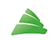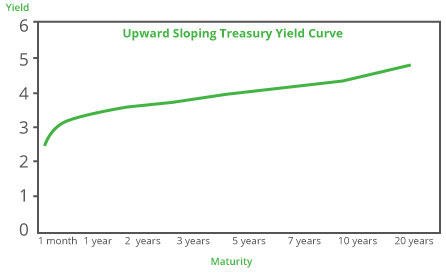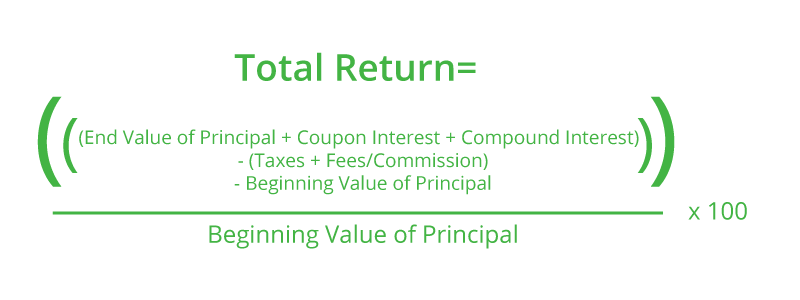# BOND YIELD AND RETURNBACK

Yield is a general term that relates to the return on the capital you invest in the bond. There are a number of types of yield and more than one way to figure return on your bond investment.

BOND YIELD AND RETURN

Yield is a general term that relates to the return on the capital you invest in a bond.

There are several definitions that are important to understand when talking about yield as it relates to bonds: coupon yield, current yield, yield-to-maturity, yield-to-call and yield-to-worst.

Coupon yield is the annual interest rate established when the bond is issued.

It’s the same as the coupon rate and is the amount of income you collect on a bond, expressed as a percentage of your original investment. If you buy a bond for \$1,000 and receive \$45 in annual interest payments, your coupon yield is 4.5 percent. This amount is figured as a percentage of the bond’s par value and will not change during the lifespan of the bond.

Current yield is the bond’s coupon yield divided by its market price. Here’s the math on a bond with a coupon yield of 4.5 percent trading at 103 (\$1,030).

> Current Yield Math Example 1

Say you check the bond’s price later, and it’s trading at 101 (\$1,010). The current yield has changed:

>Current Yield Math Example 2

If you buy a new bond at par and hold it to maturity, your current yield when the bond matures will be the same as the coupon yield.YIELDS THAT MATTER MORE

Coupon and current yield only take you so far down the path of estimating the return your bond will deliver. For one, they don’t measure the value of reinvested interest. They also aren’t much help if your bond is called early—or if you want to evaluate the lowest yield you can receive from your bond. In these cases, you need to do some more advanced yield calculations. Fortunately, there is a spate of financial calculators available—some that even estimate yield on a before- and after-tax basis. The following yields are worth knowing, and should be at your broker’s fingertips:

>Yield to maturity (YTM):

Is the overall interest rate earned by an investor who buys a bond at the market price and holds it until maturity. Mathematically, it is the discount rate at which the sum of all future cash flows (from coupons and principal repayment) equals the price of the bond. YTM is often quoted in terms of an annual rate and may differ from the bond’s coupon rate. It assumes that coupon and principal payments are made on time. It does not require dividends to be reinvested, but computations of YTM generally make that assumption. Further, it does not consider taxes paid by the investor or brokerage costs associated with the purchase.

>Yield to call (YTC):

Is figured the same way as YTM, except instead of plugging in the number of months until a bond matures; you use a call date and the bond’s call price. This calculation takes into account the impact on a bond’s yield if it is called prior to maturity and should be performed using the first date on which the issuer could call the bond.

>Yield to worst (YTW):

Is whichever of a bond’s YTM and YTC is lower. If you want to know the most conservative potential return a bond can give you—and you should know it for every callable security—then perform this comparison.

Yield reflecting broker compensation is the yield adjusted by the amount of the mark-up or commission (when you purchase) or mark-down or commission (when you sell) and other fees or charges that you are charged by your broker for its services.THREE ASSUMPTIONS

YTM and YTC are based on the following assumptions:

> You hold your bond to maturity or call date.
> You reinvest every coupon.
> All coupons are reinvested at the YTM or YTC, whichever is applicable.

Interest rates regularly fluctuate, making each reinvestment at the same rate virtually impossible. Thus, YTM and YTC are estimates only, and should be treated as such. While helpful, it’s important to realize that YTM and YTC may not be the same as a bond’s total return. Such a figure is only accurately computed when you sell a bond or when it matures.

Price and yield are inversely related: As the price of a bond goes up, its yield goes down, and vice versa.You’ve probably seen financial commentators talk about the Treasury Yield Curve when discussing bonds and interest rates. It’s a handy tool because it provides, in one simple graph, the key Treasury bond data points for a given trading day, with interest rates running up the vertical axis and maturity running along the horizontal axis.

A typical yield curve is upward sloping, meaning that securities with longer holding periods carry higher yield.> Analysis:

In the yield curve above, interest rates (and also the yield) increase as the maturity or holding period increases—yield on a 30-day T-bill is 2.55 percent, compared to 4.80 percent for a 20-year Treasury bond—but not by much. When an upward-sloping yield curve is relatively flat, it means the difference between an investor’s return from a short-term bond and the return from a long-term bond is minimal. Investors would want to weigh the risk of holding a bond for a long period (see Interest Rate Risk) versus the only moderately higher interest rate increase they would receive compared to a shorter-term bond.

Indeed, yield curves can be flatter or steeper depending on economic conditions and what the Federal Reserve Board (or the “Fed”) is doing, or what investors expect the Fed to do, with the money supply. Sometimes economic conditions and expectations create a yield curve with different characteristics. For instance, an inverted yield curve slopes downward instead of up. When this happens, short-term bonds pay more than long-term bonds. Yield curve watchers generally read this as a sign that interest rates may decline.FIGURING BOND RETURN

If you’ve held a bond over a long period of time, you might want to calculate its annual percent return, or the percent return divided by the number of years you’ve held the investment.

>>Example: A \$1,000 bond held over three years with a \$145 return has a 14.5 percent return, but a 4.83 percent annual return.

When you calculate your return, you should account for annual inflation. Calculating your real rate of return will give you an idea of the buying power your earnings will have in a given year. You can determine real return by subtracting the inflation rate from your percent return.

>>Example: An investment with 5 percent return during a year of 2 percent inflation is usually said to have a real return of 3 percent.

To figure total return, start with the value of the bond at maturity (or when you sold it) and add all of your coupon earnings and compounded interest. Subtract from this figure any taxes and any fees or commissions. Then subtract from this amount your original investment amount. This will give you the total amount of your total gain or loss on your bond investment. To figure the return as a percent, divide that number by the beginning value of your investment and multiply by 100: